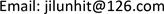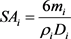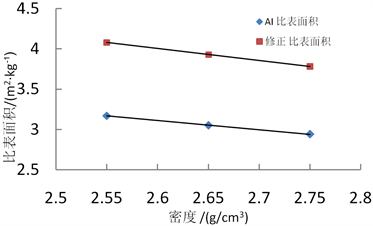﻿ 不规则颗粒–集料的比表面积分析 Analysis and Calculation Method of Specific Surface Area on Irregular Particles-Mineral Aggregate

Hans Journal of Civil Engineering
Vol.07 No.02(2018), Article ID:24209,8 pages
10.12677/HJCE.2018.72032

Analysis and Calculation Method of Specific Surface Area on Irregular Particles-Mineral Aggregate

Weigang Sun1,2, Lun Ji2, Jun Li2, Haiquan Liu2, Wendong He2,3

1Heilongjiang Ba-da Road & Bridge Construction Co., Ltd., Harbin Heilongjiang

2School of Transportation Science and Engineering, Harbin Institute of Technology, Harbin Heilongjiang

3Shanxi Transportation Research Institute, Taiyuan ShanxiReceived: Mar. 7th, 2018; accepted: Mar. 21st, 2018; published: Mar. 28th, 2018ABSTRACT

Specific surface area of irregular particle is an important indicator for studying physical properties, performance of composite materials and interaction. This research took aggregate in civil construction materials as an example, and used a method combining theoretical analysis with test study to propose a geometric model, which can use coefficient S to characterize specific surface area. The effects of density, geometric shape factor and their combined action on calculation results of specific surface area were studied. The calculation results of specific surface area obtained through modified method is larger by 30% than AI method. The maximum specific surface area coefficient difference among different aggregates can reach 2.5 times. The effect of surface texture structure on calculation of specific surface area was analyzed, and correction factor Kw of texture structure and relative correction method were proposed through characterizing surface structure feature of aggregate. The surface analysis and calculation method of specific surface area based on density, geometric shape and surface structure were proposed.

Keywords:Irregular Particles, Calculation Method of Specific Surface Are, Multi-Factor Analysis of Specific Surface Area, Shape Coefficient, Correction Factor

1黑龙江省八达路桥建设有限公司，黑龙江 哈尔滨

2哈尔滨工业大学，交通科学与工程学院，黑龙江 哈尔滨

3山西省交通科学研究院，山西 太原1. 引言

2. 不规则颗粒比表面积计算面对的问题

3. 不规则颗粒–集料比表面积的计算

3.1. 集料比表面积的计算原理分析

1) 分组

2) 颗粒形状描述

3) 计算每一组集料的比表面积系数FAi

${V}_{i}=\frac{{m}_{i}}{{\rho }_{i}}$ (1)

${s}_{i}=\frac{{V}_{i}}{{v}_{i}}$ (2)

${V}_{i}=\frac{4}{3}\text{π}{D}_{i}^{3}$ (3)

${A}_{i}=4\text{π}{\left(\frac{{D}_{i}}{2}\right)}^{2}$ (4)

$S{A}_{i}={s}_{i}×{A}_{i}=\frac{{V}_{i}}{{v}_{i}}×{A}_{i}=\frac{\frac{{m}_{i}}{{\rho }_{i}}}{\frac{4}{3}\text{π}{D}_{i}^{3}}×4\text{π}{\left(\frac{{D}_{i}}{2}\right)}^{2}$

$S{A}_{i}=\frac{12{m}_{i}}{{\rho }_{i}{D}_{i}}$ (5)

$F{A}_{i}=\frac{12}{{\rho }_{i}{D}_{i}}$ (6)

$F{A}_{i}=\frac{S}{{\rho }_{i}{D}_{i}}$ (7)

4) 计算质量为m的集料比表面积SA

$SA=\sum _{1}^{n}S{A}_{i}=\sum _{1}^{n}F{A}_{i}×{m}_{i}$ (8)

3.2. 美国沥青协会(AI)比表面积计算方法

AI的方法中各个筛孔的表面积系数，即

$F{A}_{i}=\frac{C}{{G}_{a}d}$ (9)

$SA=\sum _{i=1}^{n}\left({P}_{i}×F{A}_{i}\right)$ (10)

SA为集料的总表面积(单位，m2∙kg−1)；

Pi为集料在各个筛孔的分计筛余百分数(%，有的资料  将该参数定义为通过率)；

FAi为各个筛孔的修正表面积系数(单位，m2∙kg−1)。Table 1. AI special surface areas coefficient of each sieve

4. 不规则颗粒比表面积计算原理

4.1. 集料比表面积的计算原理分析

${v}_{i}={a}_{i}^{3}$ (11)

${A}_{i}=6{a}_{i}^{2}$ (12)(13)

$F{A}_{i}=\frac{6}{{\rho }_{i}{D}_{i}}$ (14)

${S}_{cubo}=2×\left(1+m+\frac{m}{k}\right)$ (15)

$k=m=\text{1}$ 时，即为正方体模型。该模型可以描述集料长方体形状，而更加重要的是，采用该模型可以描述和分析混合料中的针片状。表2列出了不同模型分析所得的形状系数S。

4.2. 集料的密度影响修正

AI方法对密度进行的修正，即，按照密度的比例关系进行的修正。

4.3. 集料的密度及形状耦合因素影响的修正Table 2. The shape factor (S) in different shape model coefficient of mineral aggregatesTable 4. The specific surface areas coefficient/(m2∙kg−1) and the specific area/(m2∙kg−1) considering densityTable 5. The specific surface areas coefficient/(m2∙kg−1) and the specific surface areas/(m2∙kg−1) considering the mutual coupling effects between density and shapeFigure 1. Specific surface areas considering density by various calculation methods

5. 不规则颗粒比表面积分析方法

6. 结论

Analysis and Calculation Method of Specific Surface Area on Irregular Particles-Mineral Aggregate[J]. 土木工程, 2018, 07(02): 279-286. https://doi.org/10.12677/HJCE.2018.72032

1. 1. 周云麟. 混凝土骨料比表面积的测定与计算[J]. 混凝土及加筋混凝土, 1984(2): 50-53.

2. 2. Kademli, M. (2013) Comparison of Subsieving Techniques Based on the Relation of Particle Size and Specific Surface Area in Mineral Processing. Particulate Science and Technology, 31, 326-331. https://doi.org/10.1080/02726351.2012.736457

3. 3. 王海峰, 国冬花, 刘树平. 基于颗粒特征的沥青膜厚度计算法概述[J]. 交通世界, 2009(z1): 192-193.

4. 4. 郑玉. 集料比表面积计算方法探讨[J]. 交通运输研究, 2008(21): 95-97.

5. 5. 吕得保, 刘寒冰, 关长禄. 沥青混合料的集料比表面积确定方法的研究[J]. 公路交通科技, 2011, 28(5): 28-32.

6. 6. Joseph, K. Ano-chie-Boateng, J.K. and Tutumluer, E. (2012) Aggregate Surface Areas Quantified through Laser Measurements for South African Asphalt Mixtures. Journal of Transportation Engineering, 2012, 1006-1015.

7. 7. Bhasin, A. and Little, D.N. (2006) Characterizing Surface Properties of Aggregates Used in Hot Mix Asphalt. Texas Transportation Institute Texas A&M University, College Station, 10-18.

8. 8. Lee, D.-Y., Guinn, J.A., Khandhal, P.S. and Robert, L. (1996) Absorption of Asphalt into Porous Aggregates. SHRP-A/UIR-90-009. Strategic Highway Research Program National Research Council, Washington DC.

9. 9. 李福普, 沈金安. JTG F40-2004 公路沥青路面施工技术规范[S]. 北京: 人民交通出版社, 2005: 88-89.

10. 10. Fernlund, J.M.R. (2005)3-D Image Analysis Size and Shape Method Applied to the Evaluation of the Los Angeles Test. Engineering Geology, 77, 57-67. https://doi.org/10.1016/j.enggeo.2004.08.002

11. 11. Garboczi, E.J., Cheok, G.S. and Stone, W.C. (2006) Using LADAR to Cha-racterize the 3-D Shape of Aggregates: Preliminary Results. Cement and Concrete Research, 36, 1072-1075. https://doi.org/10.1016/j.cemconres.2006.03.017

12. 12. 陈杰, 李红杰, 万成. 基于数字图像技术的集料针片状量测方法[J]. 中国公路, 2013(8): 124-125.